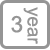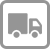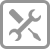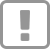• 产品详情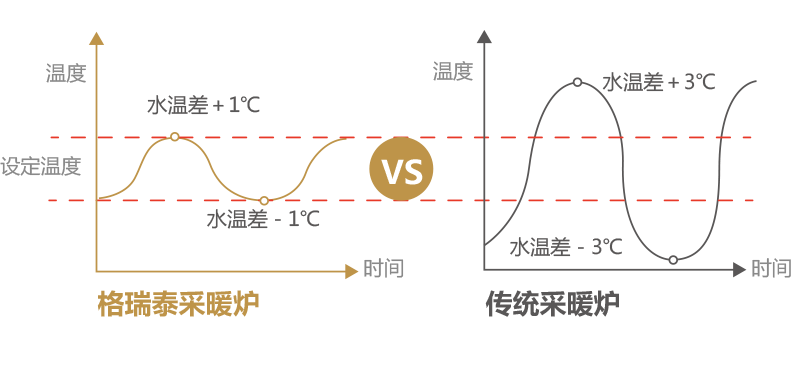^^^^^^^^^^^^^^^^^^^^^^^^^^^^^^^^^^^^^^^^^^^^^^^^^^^^^^^^^^^^^^^^^^^^^^^^^^^^^^^

• 低噪音运行。

• 安装快捷，无需另配挂板。

• 具有数码显示屏，可数字化显示采暖系统出水温度和洗浴水温度。当系统出现故障时，可自动检测并显示故障代码，便于维修查找故障原因。

• 压力表显示系统水压力，防止系统缺水。

• 3bar安全阀保护，防止管路系统超压。

• 设有自动旁通保护，在管路堵塞时可自动保护壁挂炉，避免主换热器干烧。

• 极限温度保护，防止壁挂炉过热。

• 采用同轴平衡式烟道，解决烟道朝向问题。

• 采暖系统采用闭式循环，解决了氧腐蚀问题。

• 采暖和洗浴状态自由进行切换。

• IP44绝缘保护等级，家用燃气壁挂炉可在潮湿和环境恶劣的条件下使用。

• 结构简洁，整机刚性强，空气室密封严密，燃烧安全系数高。

• 产品符合欧洲EN483标准。

• 为每一台机提供一个防尘罩，以便在装好壁挂炉或夏季不用时为壁挂炉提供良好保护。

• 设有开机后20S内升温不够3度的锁定程序，能杜绝无法检测因停电造成的管内冻结而发生恶性炸机现象。

^^^^^^^^^^^^^^^^^^^^^^^^^^^^^^^^^^^^^^^^^^^^^^^^^^^^^^^^^^^^^^^^^^^^^^^^^^^^^^^

BG系列燃气壁挂炉具有卫生热水60℃限温、采暖水85℃限温、缺水（低水压）保护、防干烧保护、风压保护、停气保护、防冻保护等十四种保护措施，安全性极佳。

1、卫生热水限温保护

2、采暖水限温保护

3、极限恒温保护

4、低水压保护

5、防干烧保护

6、水系统过压保护

7、风压保护

8、停气手动恢复保护

9、停电复位保护

10、熄火保护功能

11、预吹扫功能

12、防卡死保护

13、防冻保护

14、三分钟延时保护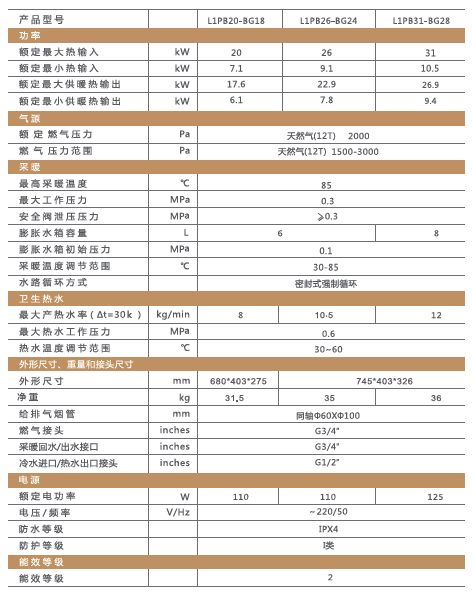>

Professional security care for a better life

【享受服务】